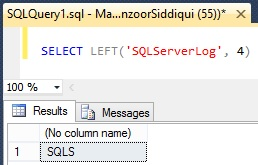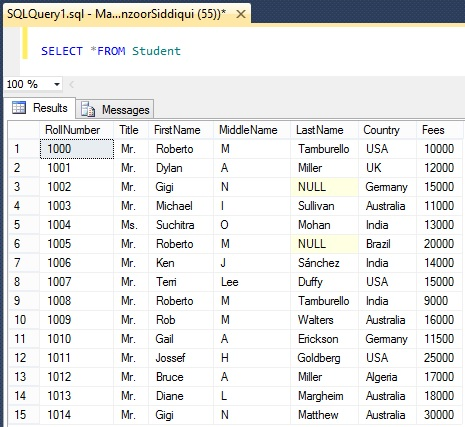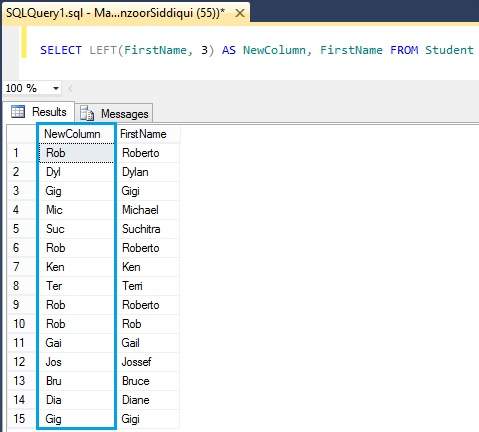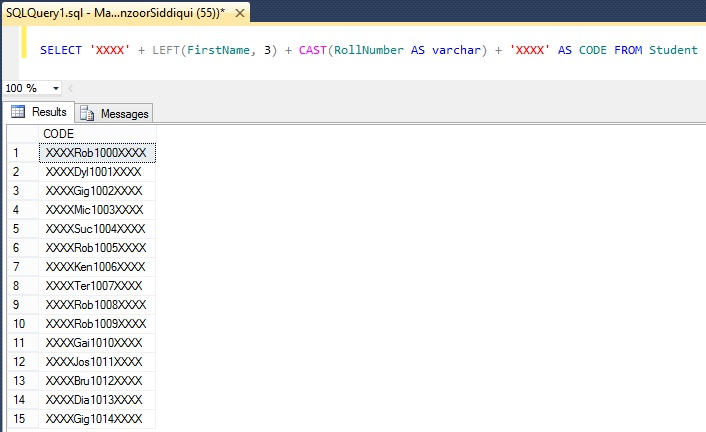# SQL LEFT() Function

SQL LEFT() function returns substring from left side of specified string from a given column. If any column cell contains NULL value then it returns output as NULL for that value.

## Syntax

SELECT LEFT (String, IntegerValue)

Below is a simple example for LEFT() function where we are selecting four characters from left side of given string.

`SELECT LEFT('SQLServerLog', 4)`Now, we will consider examples from Student table.Here we will select three characters from left side of FirstName column with below query.

`SELECT LEFT(FirstName, 3) AS NewColumn, FirstName FROM Student`As you can see, we have extracted left three characters from FirstName column with LEFT() function. Similarly you can add more columns and add paddings to represent as code. We will see one more query.

`SELECT 'XXXX' + LEFT(FirstName, 3) + CAST(RollNumber AS varchar) + 'XXXX' AS CODE FROM Student `

Here, we are selecting three characters from left side of FirstName column with RollNumber column and padding with ‘XXXX’ value on both side with new column name as CODE.Reference: Manzoor Siddiqui [www.SQLServerLog.com]